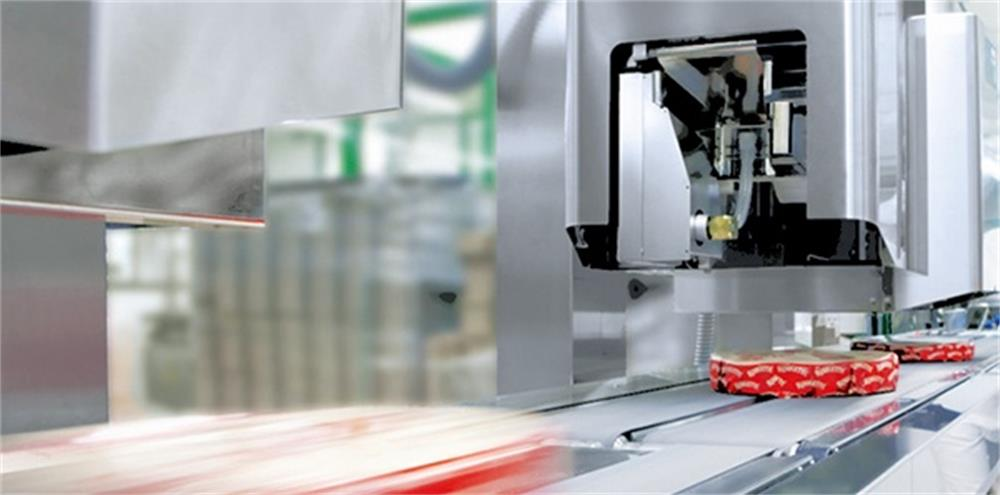How Do You Check the Accuracy of a Checkweigher Correctly?

# How Do You Check the Accuracy of a Checkweigher Correctly?

Summary

Many people will have some misunderstandings in testing the accuracy of the checkweigher, resulting in some errors in the test results. This article will specifically introduce the correct method of checking the accuracy of the checkweigher.On any weighing instrument, there are two areas for error that will determine the accuracy of the equipment. The first is the repeatability and the second is an exactness to a known mass, such as calibrated weight. These two factors are the only similarity between dynamic weighing (inline checkweigher) and static weighing (scales). The following is the test method to check the accuracy of the checkweigher.

The most common misunderstanding is that an inline checkweigher should be calibrated the same as a static scale, meaning you stop the transport system, place weights on the weighing conveyor, and if this is OK then the checkweigher is accurate.

This is not true as you have to consider the additional errors introduced when you start the conveyor and the checkweigher is working with additional vibration and in motion. This is a big factor and fundamentally only a checkweigher that has a weigh cell that is designed to work in this way will in many cases meet the required accuracy.

While static calibration as the only form of calibration is pointless, for the service engineer a starting reference should be used to determine the dynamic weight. For example, the checkweigher can weigh a product at 500g statically but when in motion the display shows 502g, the checkweigher would need to correct the dynamic by -2g. Ideally, a checkweigher’s auto dynamic calibration will adjust for this by taking a static reference first and then measuring the difference and adjusting.

The other misunderstanding is passing a known weight across the checkweigher, such as a calibration weight. This calibration weight even if it is the same weight as your product will have a different dynamic effect than your product as it is transferred across the weighing conveyor.

Doing this test will determine the repeatability of the checkweigher with the product. You should always start with the repeatability, as the other test to a known mass cannot be determined without a stable repeatable reading first.

In order to do this you should do the following; Pass the same product 10 or 20 times over the checkweigher, ensure that you pass the product in the direction it would be during production.

(1) Record these individual values, on some checkweighers there is a function to automatically record and even display the required standard deviation. If not excel has a built-in formula to do this called STDEV.

(2) From the table calculate your TNE (-T1), for example, if your product is 200g then your TNE (-T1) is 5% or 9g.

(3) You should now calculate a quarter (0.25) of this 9g, 0.25 of 9g = 2.25g. This value is your repeatability limit.

(4) If the Zone of indecision is greater than 2.25g (a quarter of TNE) then the checkweigher cannot be used with the setpoints, (reject limits) shown in the table.

This test should be done and recorded for all product types and weights used on that checkweigher. It is good practice to repeat this test over a period and build up a trend analysis, this will indicate how variable it is over time, and will then determine how frequently you will need to perform this test in the future.

The second test is for the exactness to a known mass, which is also done using the product along with a static scale, calibrated to national standards. The static scale should be capable of reading to the same resolution as the checkweigher or better.

These checks should be done before, during, and after a batch. By recording the weights a trend analysis can be made which can form the data for a risk assessment as required in some of the codes of practice.

Note the weight of a pack as it passes over the checkweigher and then weighs this on the static scale, if the difference is within 1/5 (1 fifth) TNE then the checkweigher is suitable to be used. Some checkweighers have a built-in weight check function and will reject a product and hold the weight of that product on the screen.

In order to make a check on the repeatability of the checkweigher at that time, it is good practice to repeat this test at least once.

For example, we have a product with a nominal of 200g, when we weigh this on the checkweigher it reads 200.4g. Weighing this same product on a static scale, it shows 199.7g a difference is 0.7g. We know our TNE for a 200g product is 9g and one-fifth of this is 1.8g. The 0.7g is less than the 1.8g limit therefore this checkweigher is accurate enough to comply.